Скачать презентацию Bayesian Learning Provides practical learning algorithms

b9f497ee86f912ee79eb8aeac738c633.ppt

• Количество слайдов: 25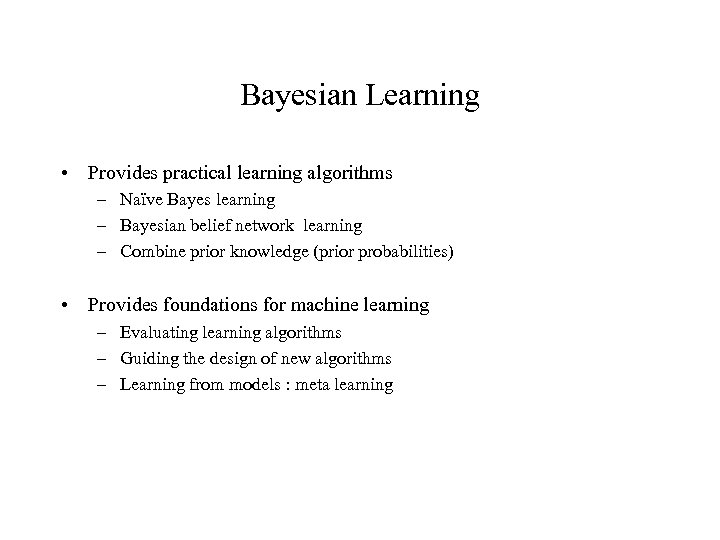Bayesian Learning • Provides practical learning algorithms – Naïve Bayes learning – Bayesian belief network learning – Combine prior knowledge (prior probabilities) • Provides foundations for machine learning – Evaluating learning algorithms – Guiding the design of new algorithms – Learning from models : meta learning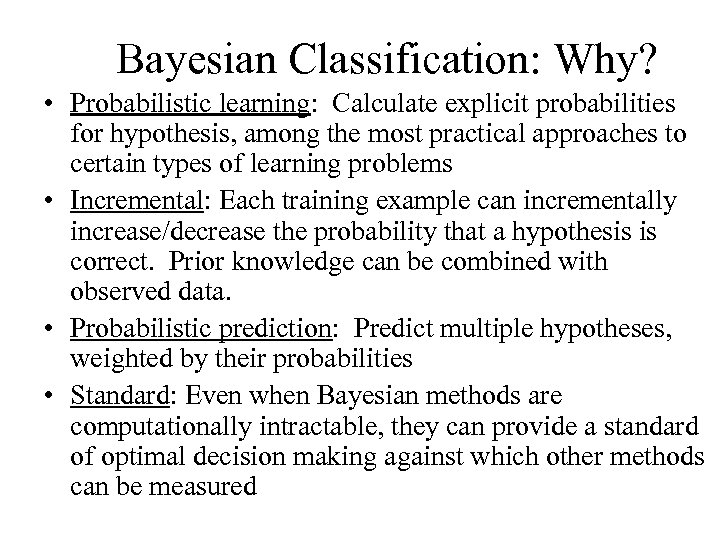Bayesian Classification: Why? • Probabilistic learning: Calculate explicit probabilities for hypothesis, among the most practical approaches to certain types of learning problems • Incremental: Each training example can incrementally increase/decrease the probability that a hypothesis is correct. Prior knowledge can be combined with observed data. • Probabilistic prediction: Predict multiple hypotheses, weighted by their probabilities • Standard: Even when Bayesian methods are computationally intractable, they can provide a standard of optimal decision making against which other methods can be measured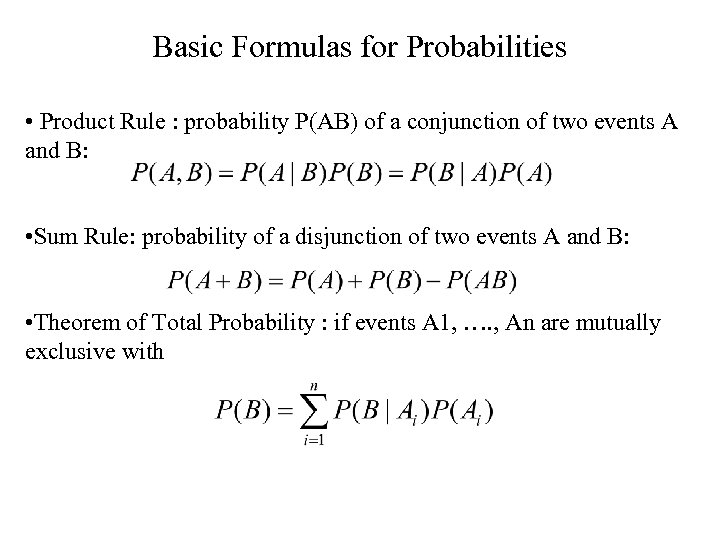Basic Formulas for Probabilities • Product Rule : probability P(AB) of a conjunction of two events A and B: • Sum Rule: probability of a disjunction of two events A and B: • Theorem of Total Probability : if events A 1, …. , An are mutually exclusive with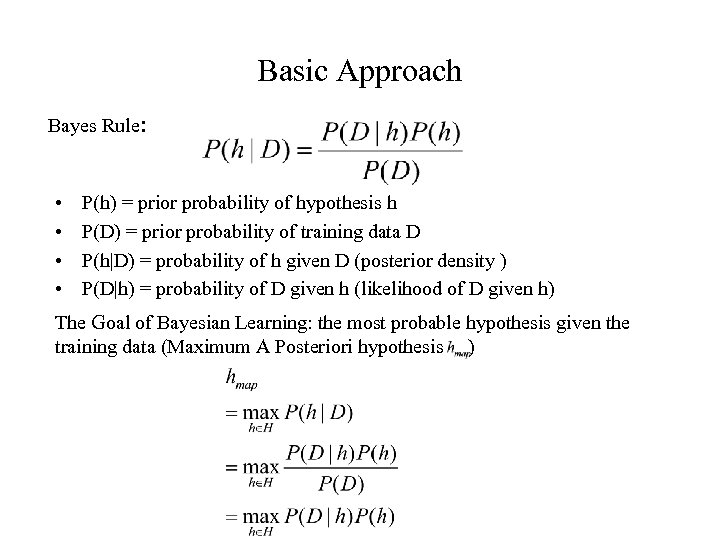Basic Approach Bayes Rule: • • P(h) = prior probability of hypothesis h P(D) = prior probability of training data D P(h|D) = probability of h given D (posterior density ) P(D|h) = probability of D given h (likelihood of D given h) The Goal of Bayesian Learning: the most probable hypothesis given the training data (Maximum A Posteriori hypothesis )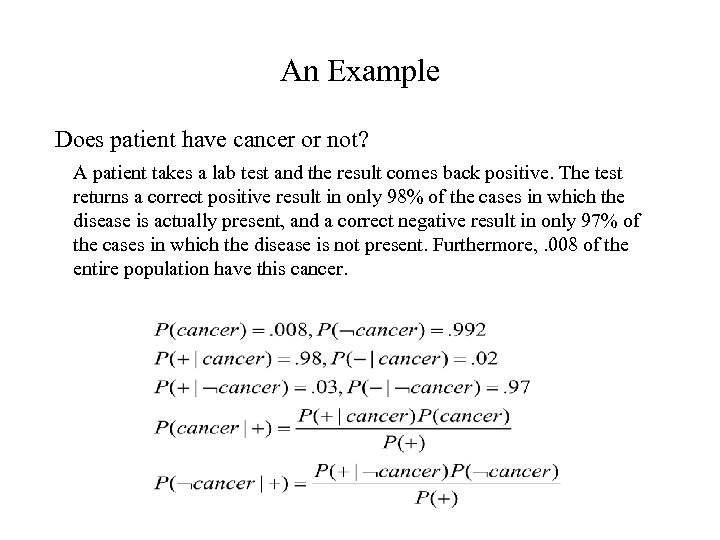An Example Does patient have cancer or not? A patient takes a lab test and the result comes back positive. The test returns a correct positive result in only 98% of the cases in which the disease is actually present, and a correct negative result in only 97% of the cases in which the disease is not present. Furthermore, . 008 of the entire population have this cancer.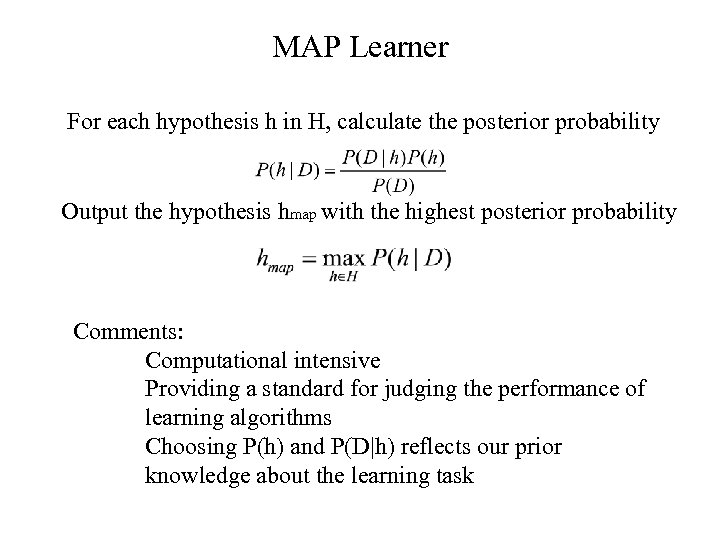MAP Learner For each hypothesis h in H, calculate the posterior probability Output the hypothesis hmap with the highest posterior probability Comments: Computational intensive Providing a standard for judging the performance of learning algorithms Choosing P(h) and P(D|h) reflects our prior knowledge about the learning task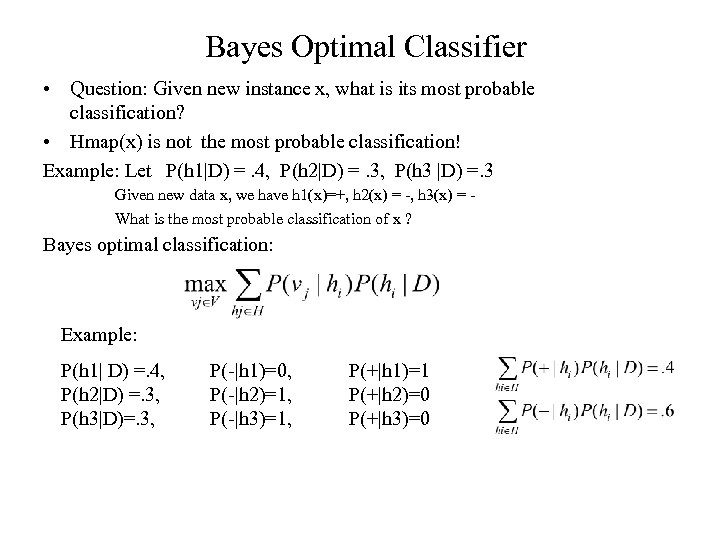Bayes Optimal Classifier • Question: Given new instance x, what is its most probable classification? • Hmap(x) is not the most probable classification! Example: Let P(h 1|D) =. 4, P(h 2|D) =. 3, P(h 3 |D) =. 3 Given new data x, we have h 1(x)=+, h 2(x) = -, h 3(x) = What is the most probable classification of x ? Bayes optimal classification: Example: P(h 1| D) =. 4, P(h 2|D) =. 3, P(h 3|D)=. 3, P(-|h 1)=0, P(-|h 2)=1, P(-|h 3)=1, P(+|h 1)=1 P(+|h 2)=0 P(+|h 3)=0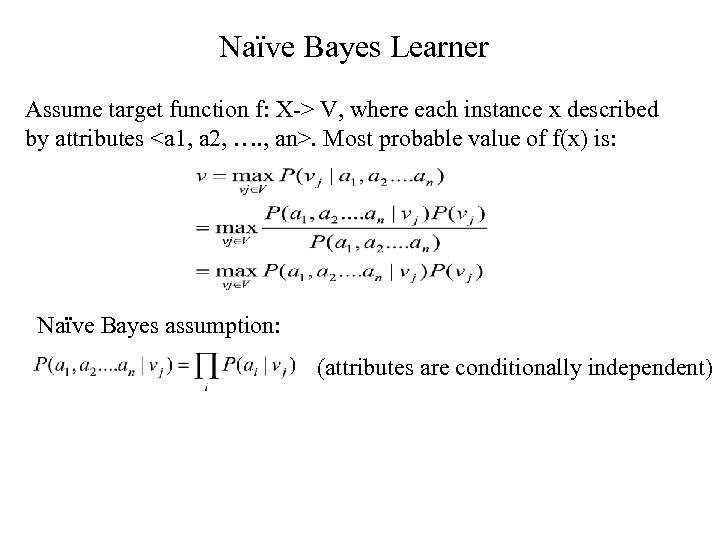Naïve Bayes Learner Assume target function f: X-> V, where each instance x described by attributes . Most probable value of f(x) is: Naïve Bayes assumption: (attributes are conditionally independent)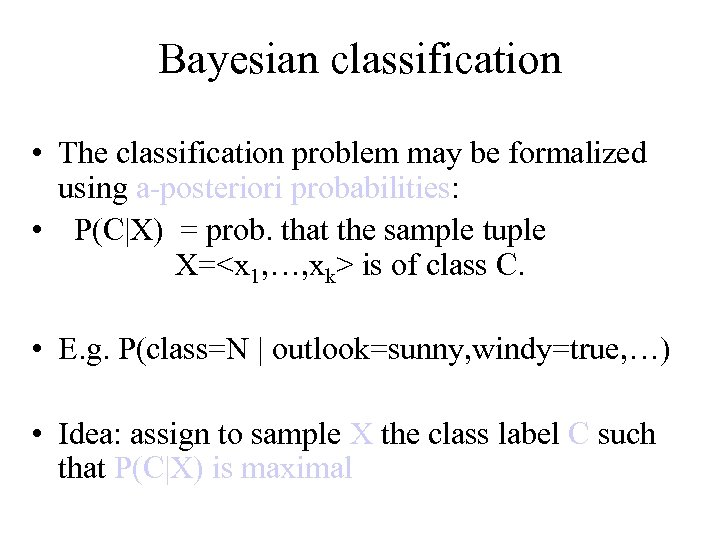Bayesian classification • The classification problem may be formalized using a-posteriori probabilities: • P(C|X) = prob. that the sample tuple X= is of class C. • E. g. P(class=N | outlook=sunny, windy=true, …) • Idea: assign to sample X the class label C such that P(C|X) is maximal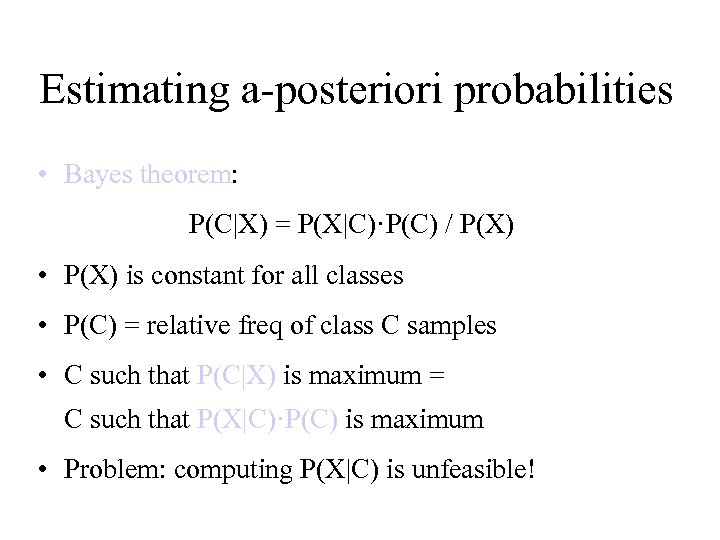Estimating a-posteriori probabilities • Bayes theorem: P(C|X) = P(X|C)·P(C) / P(X) • P(X) is constant for all classes • P(C) = relative freq of class C samples • C such that P(C|X) is maximum = C such that P(X|C)·P(C) is maximum • Problem: computing P(X|C) is unfeasible!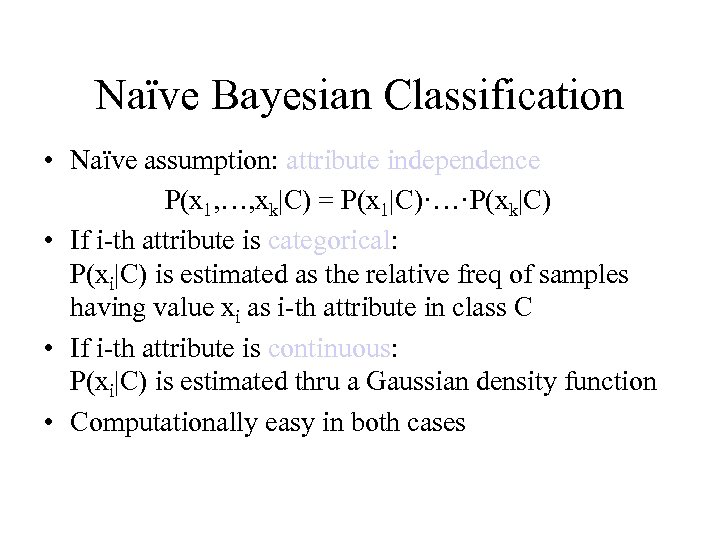Naïve Bayesian Classification • Naïve assumption: attribute independence P(x 1, …, xk|C) = P(x 1|C)·…·P(xk|C) • If i-th attribute is categorical: P(xi|C) is estimated as the relative freq of samples having value xi as i-th attribute in class C • If i-th attribute is continuous: P(xi|C) is estimated thru a Gaussian density function • Computationally easy in both cases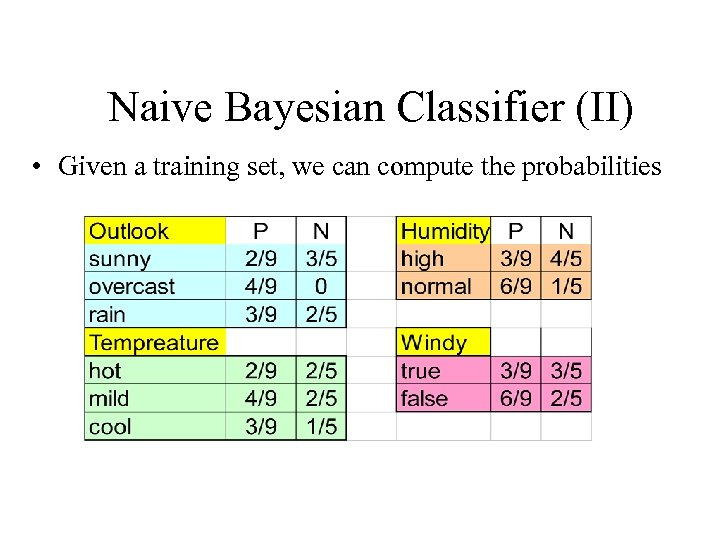Naive Bayesian Classifier (II) • Given a training set, we can compute the probabilities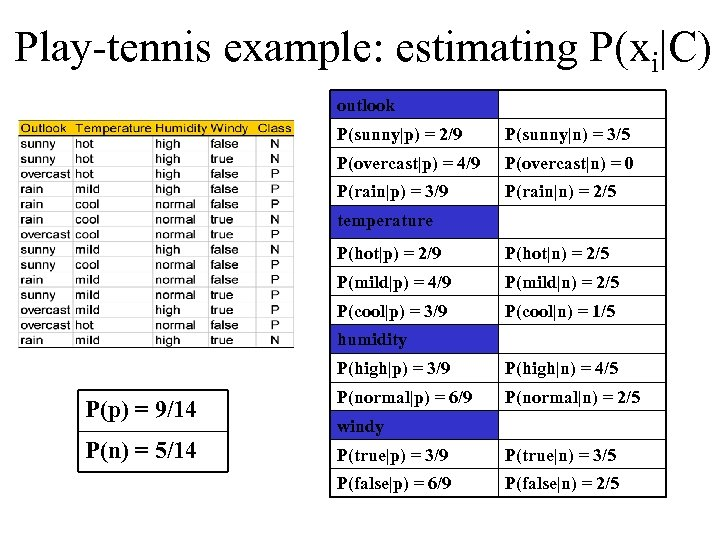Play-tennis example: estimating P(xi|C) outlook P(sunny|p) = 2/9 P(sunny|n) = 3/5 P(overcast|p) = 4/9 P(overcast|n) = 0 P(rain|p) = 3/9 P(rain|n) = 2/5 temperature P(hot|p) = 2/9 P(hot|n) = 2/5 P(mild|p) = 4/9 P(mild|n) = 2/5 P(cool|p) = 3/9 P(cool|n) = 1/5 humidity P(high|p) = 3/9 P(high|n) = 4/5 P(p) = 9/14 P(normal|p) = 6/9 P(normal|n) = 2/5 P(n) = 5/14 P(true|p) = 3/9 P(true|n) = 3/5 P(false|p) = 6/9 P(false|n) = 2/5 windy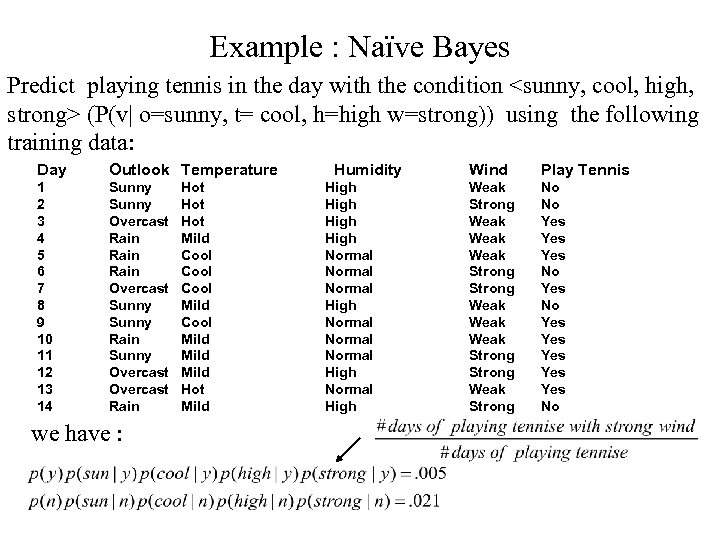Example : Naïve Bayes Predict playing tennis in the day with the condition (P(v| o=sunny, t= cool, h=high w=strong)) using the following training data: Day Outlook Temperature 1 2 3 4 5 6 7 8 9 10 11 12 13 14 Sunny Overcast Rain Overcast Sunny Rain Sunny Overcast Rain we have : Hot Hot Mild Cool Mild Hot Mild Humidity High Normal Normal High Wind Play Tennis Weak Strong Weak Weak Strong Weak Strong No No Yes Yes Yes No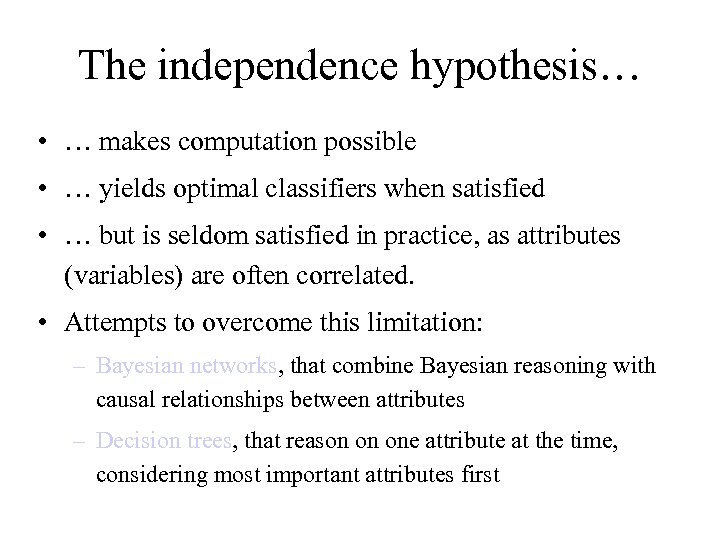The independence hypothesis… • … makes computation possible • … yields optimal classifiers when satisfied • … but is seldom satisfied in practice, as attributes (variables) are often correlated. • Attempts to overcome this limitation: – Bayesian networks, that combine Bayesian reasoning with causal relationships between attributes – Decision trees, that reason on one attribute at the time, considering most important attributes first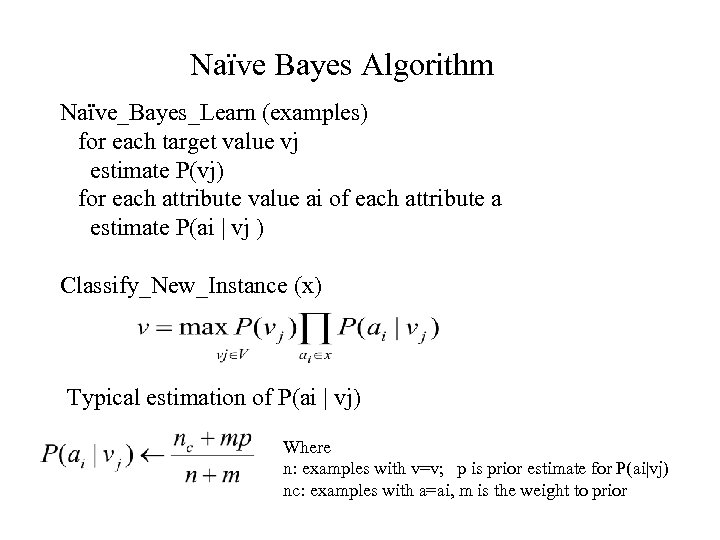Naïve Bayes Algorithm Naïve_Bayes_Learn (examples) for each target value vj estimate P(vj) for each attribute value ai of each attribute a estimate P(ai | vj ) Classify_New_Instance (x) Typical estimation of P(ai | vj) Where n: examples with v=v; p is prior estimate for P(ai|vj) nc: examples with a=ai, m is the weight to prior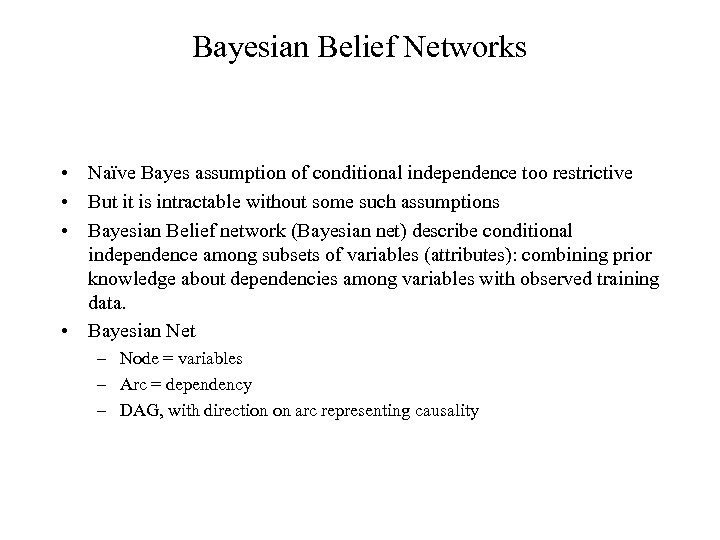Bayesian Belief Networks • Naïve Bayes assumption of conditional independence too restrictive • But it is intractable without some such assumptions • Bayesian Belief network (Bayesian net) describe conditional independence among subsets of variables (attributes): combining prior knowledge about dependencies among variables with observed training data. • Bayesian Net – Node = variables – Arc = dependency – DAG, with direction on arc representing causality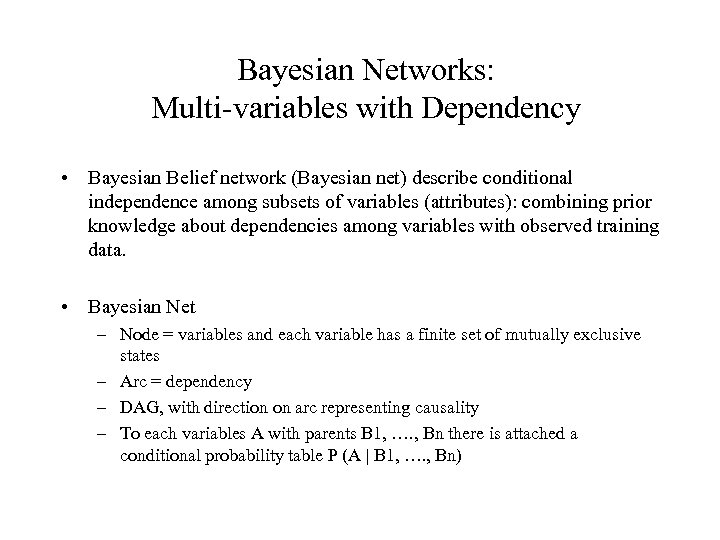Bayesian Networks: Multi-variables with Dependency • Bayesian Belief network (Bayesian net) describe conditional independence among subsets of variables (attributes): combining prior knowledge about dependencies among variables with observed training data. • Bayesian Net – Node = variables and each variable has a finite set of mutually exclusive states – Arc = dependency – DAG, with direction on arc representing causality – To each variables A with parents B 1, …. , Bn there is attached a conditional probability table P (A | B 1, …. , Bn)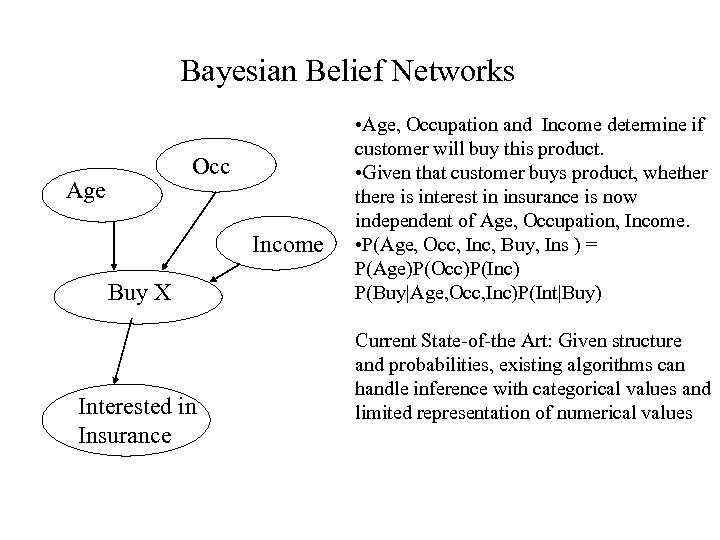Bayesian Belief Networks Occ Age Income Buy X Interested in Insurance • Age, Occupation and Income determine if customer will buy this product. • Given that customer buys product, whethere is interest in insurance is now independent of Age, Occupation, Income. • P(Age, Occ, Inc, Buy, Ins ) = P(Age)P(Occ)P(Inc) P(Buy|Age, Occ, Inc)P(Int|Buy) Current State-of-the Art: Given structure and probabilities, existing algorithms can handle inference with categorical values and limited representation of numerical values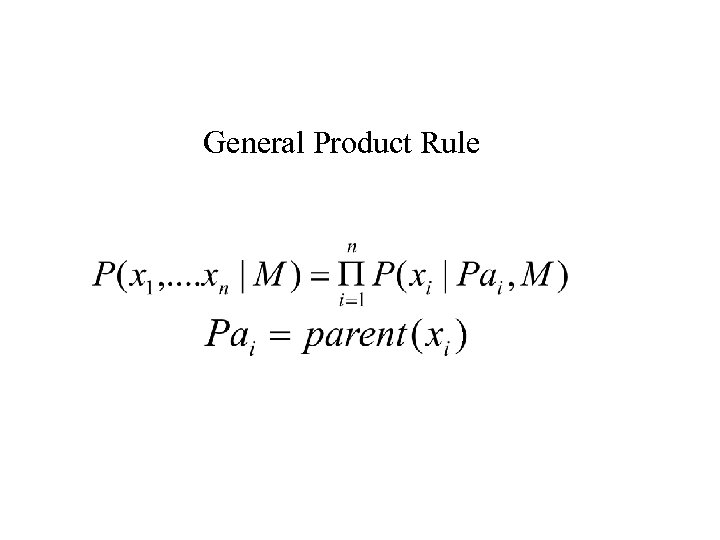General Product Rule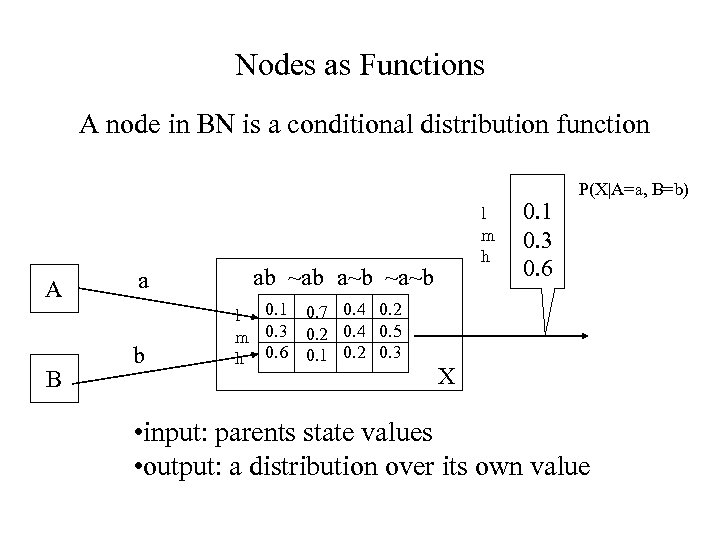Nodes as Functions A node in BN is a conditional distribution function A B a b l m h ab ~ab a~b ~a~b l 0. 1 m 0. 3 h 0. 6 0. 7 0. 4 0. 2 0. 4 0. 5 0. 1 0. 2 0. 3 0. 1 0. 3 0. 6 P(X|A=a, B=b) X • input: parents state values • output: a distribution over its own value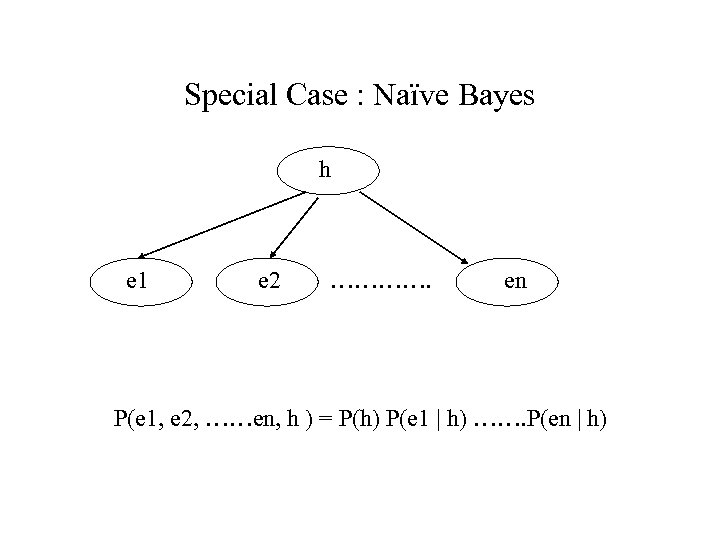Special Case : Naïve Bayes h e 1 e 2 …………. en P(e 1, e 2, ……en, h ) = P(h) P(e 1 | h) ……. P(en | h)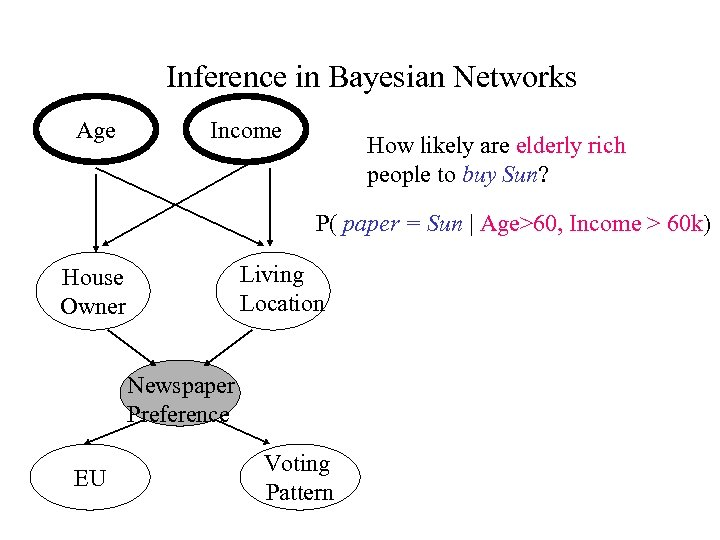Inference in Bayesian Networks Age Income How likely are elderly rich people to buy Sun? P( paper = Sun | Age>60, Income > 60 k) Living Location House Owner Newspaper Preference EU Voting Pattern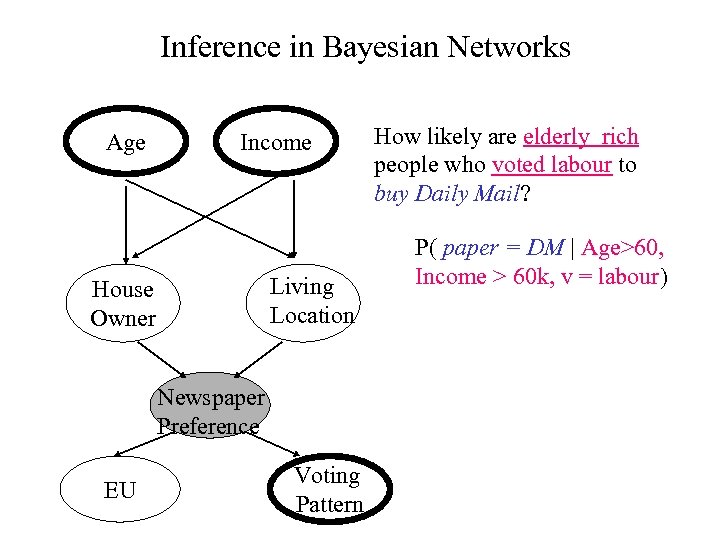Inference in Bayesian Networks Age Income Living Location House Owner Newspaper Preference EU Voting Pattern How likely are elderly rich people who voted labour to buy Daily Mail? P( paper = DM | Age>60, Income > 60 k, v = labour)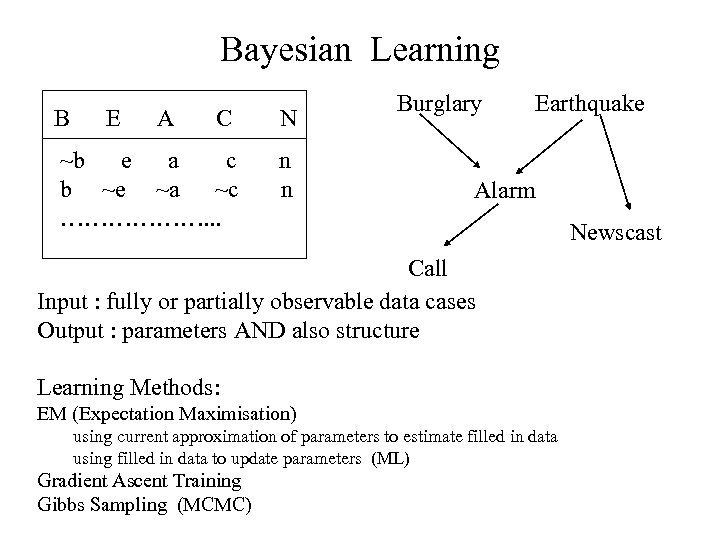Bayesian Learning B E A C N ~b e a c b ~e ~a ~c ………………. . . n n Burglary Earthquake Alarm Call Input : fully or partially observable data cases Output : parameters AND also structure Learning Methods: EM (Expectation Maximisation) using current approximation of parameters to estimate filled in data using filled in data to update parameters (ML) Gradient Ascent Training Gibbs Sampling (MCMC) Newscast